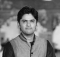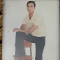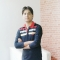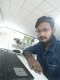## How to fetch a specific row when values are the same in MySQL?Published on 25-Aug-2019 06:10:00
To fetch a specific row when values are the same, use GROUP BY. Let us first create a table −mysql> create table DemoTable659(Id int, Name varchar(100), Score int); Query OK, 0 rows affected (0.52 sec)Insert some records in the table using insert command −mysql> insert into DemoTable659 values(11, 'John', 45); ... Read More

## Mathematical Logical ConnectivesPublished on 25-Aug-2019 06:10:00
A Logical Connective is a symbol which is used to connect two or more propositional or predicate logics in such a manner that resultant logic depends only on the input logics and the meaning of the connective used.Generally there are five connectives which are −OR (∨)AND (∧)Negation/ NOT (¬)Implication / ... Read More

## exec() in PythonPublished on 25-Aug-2019 06:10:00
Exec function can dynamically execute code of python programs. The code can be passed in as string or object code to this function. The object code is executed as is while the string is first parsed and checked for any syntax error. If no syntax error, then the parsed string ... Read More

## Matching GraphPublished on 25-Aug-2019 06:10:00
A matching graph is a subgraph of a graph where there are no edges adjacent to each other. Simply, there should not be any common vertex between any two edges.MatchingLet 'G' = (V, E) be a graph. A subgraph is called a matching M(G), if each vertex of G is ... Read More

## degrees() and radians() in PythonPublished on 25-Aug-2019 06:10:00
The measurements of angles in mathematics are done using these two units of measurement called degree and radian. They are frequently used in math calculations involving angles and need conversion from one value to another. In python we can achieve these conversions using python functions.degrees() FunctionThis function takes radian value ... Read More

## How to convert a MySQL TIME value to days and hours form?Published on 25-Aug-2019 06:10:00
Here, we are converting time value, for example 150:50:10 to days and hours form, i.e. 6 days 6 hours.You can use CONCAT() along with HOUR() for this. Let us first create a table −mysql> create table DemoTable657(DueTime time); Query OK, 0 rows affected (3.68 sec)Insert some records in the table ... Read More

## Line/Edge CoveringPublished on 25-Aug-2019 06:10:00
A covering graph is a subgraph which contains either all the vertices or all the edges corresponding to some other graph. A subgraph which contains all the vertices is called a line/edge covering. A subgraph which contains all the edges is called a vertex covering.Line CoveringLet G = (V, E) ... Read More

## JavaScript Sleep() function?Published on 25-Aug-2019 06:10:00
Sleep()With the help of Sleep() we can make a function to pause execution for a fixed amount of time. In programming languages such as C and Php we would call sleep(sec). Java has thread.sleep(), python has time.sleep() and GO has time.Sleep(2 * time.Second).javascript doesn't have these kinds of sleep functions. But we should thank promises and ... Read More

## Counting the frequencies in a list using dictionary in PythonPublished on 25-Aug-2019 06:10:00
In this article we develop a program to calculate the frequency of each element present in a list.Using a dictionaryHere we capture the items as the keys of a dictionary and their frequencies as the values.Example Live Demolist = ['a', 'b', 'a', 'c', 'd', 'c', 'c'] frequency = {} for item ... Read More

## Kirchoff's TheoremPublished on 25-Aug-2019 06:10:00
Kirchoff's theorem is useful in finding the number of spanning trees that can be formed from a connected graph.ExampleThe matrix 'A' be filled as, if there is an edge between two vertices, then it should be given as '1', else '0'.
Advertisements